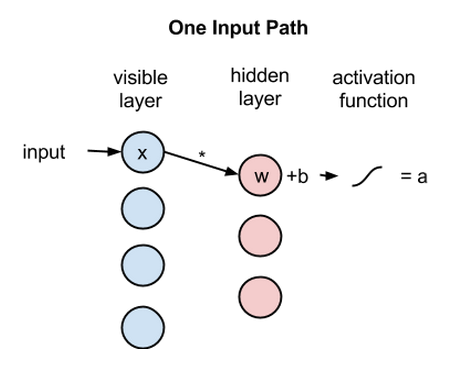/  Deep Learning Interview questions and answers   /  What is Restricted Boltzmann Machine?## What is Restricted Boltzmann Machine?

A Restricted Boltzmann machine is an algorithm useful for dimensionality reduction, classification, regression, collaborative filtering, feature learning and topic modeling. RBMs are shallow, two-layer neural nets that constitute the building blocks of deep-belief networks. The first layer of the RBM is called the visible, or input, layer, and the second is the hidden layer. There is no intra-layer communication – this is the restriction in a restricted Boltzmann machine. Each node is a locus of computation that processes input, and begins by making stochastic decisions about whether to transmit that input or not.

Each visible node takes a low-level feature from an item in the dataset to be learned. At node 1 of the hidden layer, x is multiplied by a weight and added to a so-called bias. The result of those two operations is fed into an activation function, which produces the node’s output, or the strength of the signal passing through it, given input x.

activation f((weight w * input x) + bias b ) = output aNext, let’s look at how several inputs would combine at one hidden node. Each x is multiplied by a separate weight, the products are summed, added to a bias, and again the result is passed through an activation function to produce the node’s output.Because inputs from all visible nodes are being passed to all hidden nodes, an RBM can be defined as a symmetrical bipartite graph.

Symmetrical means that each visible node is connected with each hidden node (see below). Bipartite means it has two parts, or layers, and the graph is a mathematical term for a web of nodes.

At each hidden node, each input x is multiplied by its respective weight w. That is, a single input x would have three weights here, making 12 weights altogether (4 input nodes x 3 hidden nodes). The weights between two layers will always form a matrix where the rows are equal to the input nodes, and the columns are equal to the output nodes.

Each hidden node receives the four inputs multiplied by their respective weights. The sum of those products is again added to a bias (which forces at least some activations to happen), and the result is passed through the activation algorithm producing one output for each hidden node.If these two layers were part of a deeper neural network, the outputs of hidden layer no. 1 would be passed as inputs to hidden layer no. 2, and from there through as many hidden layers as you like until they reach a final classifying layer. (For simple feed-forward movements, the RBM nodes function as an autoencoder and nothing more.)